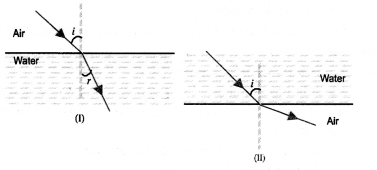# NCERT Solutions for Class 10 Science Chapter 10 Light Reflection and Refraction

NCERT Solutions for Class 10 Science Chapter 10 Light Reflection and Refraction

### NCERT Solutions for Class 10 Science Chapter 10 Intext Questions

Page Number: 168

Question 1
Define the principal focus of a concave mirror.
The principal focus of a concave mirror is a point on its principal axis to which all the light rays which are parallel and close to the axis, converge after reflection from the concave mirror.

Question 2
The radius of curvature of a spherical mirror is 20 cm. What is its focal length?
Focal length = 12 x Radius of curvature = 12 x 20 cm = 10 cm

Question 3
Name a mirror that can give an erect and enlarged image of an object.
Concave mirror.

Question 4
Why do we prefer a convex mirror as a rear-view mirror in vehicles ?
We prefer a convex mirror as a rear-view mirror in vehicles because of two reasons :

1.  A convex mirror always produces an erect image of the objects.
2.  The image formed in a convex mirror is highly diminished or much smaller than the object, due to which a convex mirror gives a wide field of view of the traffic behind. A convex mirror enables the driver to view such larger area of the traffic behind him.

Page Number: 171

Question 1
Find the focal length of a convex mirror whose radius of curvature is 32 cm.
Solution:
R = +32 cm and f=R/2=+32/2=+16cm

Question 2
A concave mirror produces three times magnified (enlarged) real image of an object placed at 10 cm in front of it. Where is the image located ?
Solution:
Because the image is real, so magnification m must be negative.Thus the image is located at a distance of 30 cm from the mirror on the object side of the mirror.

Page Number: 176

Question 1
A ray of light travelling in air enters obliquely into water. Does the light ray bend towards the normal or away from the normal ? Why ?
The light-ray bends towards the normal because the ray of light goes from a rarer medium to a denser medium.

Question 2
Light enters from air to glass having refractive index 1.50. What is the speed of light in the glass ? The speed of light in vacuum is 3 x 108 ms-1.
Solution:
Refractive index of glass, n8 = 1.50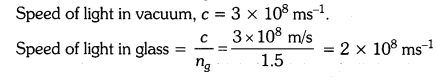Question 3
Find out, from Table 10.3, the medium having highest optical density. Also find the medium with lowest optical density.
From table 10.3, diamond has highest refractive index (= 2.42), so it has highest optical density.
Air has lowest refractive index (= 1.0003),
so it has lowest optical density.

Question 4
You are given kerosene, turpentine and water. In which of these does the light travel fastest ? Use the information given in Table 10.3.
For kerosene, n = 1.44
For turpentine, n = 1.47
For water, n = 1.33
Because water has the lowest refractive index, therefore light travels fastest in this optically rarer medium than kerosene and turpentine oil.

Question 5
The refractive index of diamond is 2.42. What is the meaning of this statement?
By saying that the refractive index of diamond is 2.42, we mean that the speed of light in diamond is lower by a factor of 2.42 relative to that in vacuum.

Page Number: 184

Question 1
Define 1 dioptre of power of a lens.
One dioptre is the power of a lens whose focal length is 1 metre.

Question 2
A convex lens forms a real and inverted image of a needle at a distance of 50 cm from it. Where is the needle placed in front of the convex lens if the image is equal to the size of the object ? Also, find the power of the lens. , Sol. Here, u — +50 cm ..
Solution:
Here ν = +50cm
Because the real image is of the same size as the object,Question 3
Find the power of a concave lens of focal length 2 m.
Solution:
Because the focal length of a concave lens is negative,
therefore f = -2 m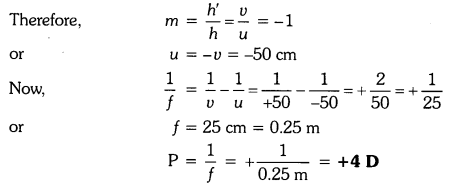### NCERT Solutions for Class 10 Science Chapter 10 Textbook Chapter End Questions

Question 1
Which one of the following materials cannot be used to make a lens ?
(a) Water
(b) Glass
(c) Plastic
(d) Clay
(d) Clay

Question 2
The image formed by a concave mirror is observed to be virtual, erect and larger than the object. Where should be the position of the object ?
(a) Between the principal focus and the centre of curvature
(b) At the centre of curvature
(c) Beyond the centre of curvature
(d) Between the pole of the mirror and its principal focus.
(d) Between the pole of the mirror and its principal focus.

Question 3
Where should an object be placed in front of a convex lens to get a real image of the size of the object ?
(a) At the principal focus of the lens (b) At twice the focal length
(c) At infinity
(d) Between the optical centre of the lens and its principal focus.
(b) At twice the focal length.

Question 4
A spherical mirror and a thin spherical lens have each a focal length of -15 cm. The mirror and the lens are likely to be :
(a) Both concave.
(b) Both convex.
(c) the mirror is concave and the lens is convex.
(d) the mirror is convex, but the lens is concave.
(a) Both concave

Question 5
No matter how far you stand from mirror, your image appears erect. The mirror is likely to be
(a) plane
(b) concave
(c) convex
(d) either plane or convex.
(d) Either plane or convex.

Question 6
Which of the following lenses would you prefer to use while reading small letters found in a dictionary ?
(a) A convex lens of focal length 50 cm.
(b) A concave lens of focal length 50 cm.
(c) A convex lens of focal length 5 cm.
(d) A concave lens of focal length 5 cm.
(c) A convex lens of focal length 5 cm.

Question 7
We wish to obtain an erect image of an object, using a concave mirror of focal length 15 cm. What should be the range of distance of the object from the mirror ? What is the nature of the image ? Is the image larger or smaller than the object ? Draw a ray diagram to show the image formation in this case.
A concave mirror gives an erect image when the object is placed between the focus F and the pole P of the concave mirror, i.e., between 0 and 15 cm from the mirror. The image thus formed will be virtual, erect and larger than the object.Question 8
Name the type of mirror used in the following situations.
(b) Side/rear-view mirror of a vehicle.
(c) Solar furnace.
(a) Concave mirrors are used as reflectors in headlights of cars. When a bulb is located at the focus of the concave mirror, the light rays after reflection from the mirror travel over a large distance as a parallel beam of high intensity.

(b) A convex mirror is used as a side/rear-view mirror of a vehicle because

• A convex mirror always forms an erect, virtual and diminished image of an object placed anywhere in front it.
• A convex mirror has a wider field of view than a plane mirror of the same size.

(c) Large concave mirrors are used to concentrate sunlight to produce heat in solar furnaces.

Question 9
One-half of a convex lens is covered with a black paper. Will this lens produce a complete image of the object ? Verify your answer experimentally. Explain your observations.
A convex lens forms complete image of an object, even if its one half is covered with black paper. It can be explained by considering following two cases.
Case I : When the upper half of the lens is covered
In this case, a ray of light coming from the object will be refracted by the lower half of the lens. These rays meet at the other side of the lens to form the image of the given object, as shown in the following figure.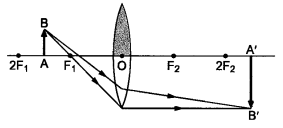Case II: When the lower half of the lens Is covered
In this case, a ray of light coming from the object is refracted by the upper half of the lens. These rays meet at the other side of the lens to form the image of the given object, as shown in the given figure.Question 10
An object 5 cm in length is held 25 cm away from a converging lens of focal length 10 cm. Draw the ray diagram and find the position, size and the nature of the image formed.
Here : Object distance, u= -25 cm,
Object height, h = 5 cm,
Focal length, f = +10 cm
According to the lens formula, 1/f=1/ν1/u , we have
⇒ 1/ν=1/f1/u=1/101/25=15/250orν=250/15=16.66cm
The positive value of v shows that the image is formed at the other side of the lens.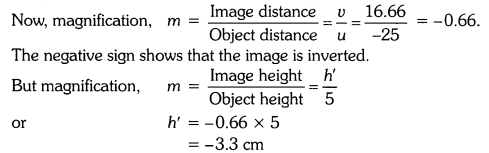The negative value of image height indicates that the image formed is inverted.
The position, size, and nature of image are shown alongside in the ray diagram.Question 11
A concave lens of focal length 15 cm forms an image 10 cm from the lens. How far is the object placed from the lens ? Draw the ray diagram.
Solution:
Focal length, f = -15 cm, Image distance, ν = -10 cm (as concave lens forms the image on the same side of the lens)
From the lens formula 1/f=1/ν1/u , we have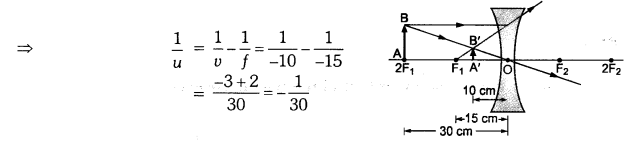Object distance, u = -30 cm
The negative value of u indicates that the object is placed in front of the lens.

Question 12
An object is placed at a distance of 10 cm from a convex mirror of focal length 15 cm. Find the position and nature of the image.
Solution:
Object distance, u = -10 cm, Focal length, f = +15 cm, Image distance, ν = ?Thus, image distance, ν = + 6 cm
Because ν is +ve, so a virtual image is formed at a distance of 6 cm behind the mirror.
Magnification, m=υ/u=6/30=1/5 (i.e. < 1)
The positive value of m shows that image erect and its value, which is less than 1, shows that image is smaller than the object. Thus, image is virtual, erect and diminished.

Question 13
The magnification produced by a plane mirror is +1. What does this mean ?
Since magnification, m=h‘/h=ν/u. Given, m = +1, so h’ = h and ν = -u

(i) m = 1 indicates the size of image is same as that of object.
(ii) positive sign of m indicates that an erect image is formed.

The opposite signs of ν and u indicate that image is formed on the other side of the mirror from where the object is placed i.e., image is formed behind the mirror and thus image formed is virtual.

Question 14
An object 5.0 cm in length is placed at a distance of 20 cm in front of a convex mirror of radius of curvature 30 cm. Find the position of the image, its nature and size.
Solution:
Since object size, h = +5 cm,
object distance, u = -20 cm
and radius of curvature, R = +30 cm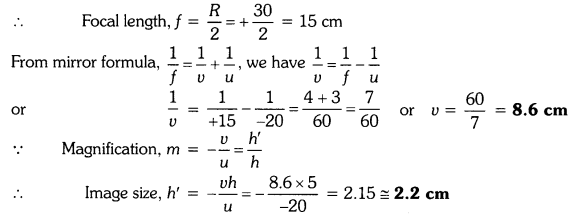A virtual, erect image of height 2.2 cm is formed behind the mirror at a distance of 8.6 cm from the mirror.

Question 15
An object of size 7.0 cm is placed at 27 cm in front of a concave mirror of focal length 18 cm. At what distance from the mirror should a screen be placed, so that a sharp focussed image can be obtained ? Find the size and the nature of the image.
Here, object size, h = +7.0 cm,
object distance, u = -27 cm
and focal length, f = -18 cm
Image distance, ν = ?
and image size, h’ = ?
From the mirror formula, 1/f=1/ν1/u, we have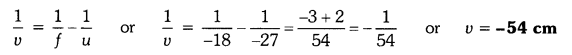The screen should be placed at a distance of 54 cm on the object side of the mirror to obtain a sharp image.The image is real, inverted and enlarged in size.

Question 16
Find the focal length of a lens of power -2.0 D. What type of lens is this ?
Here, P = -2.0 D
The type of lens is concave because the focal length is negative.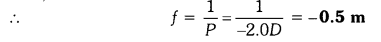Question 17
A doctor has prescribed a corrective lens of power +1.5 D. Find the focal length of the lens. Is the prescribed lens diverging or converging ?
Here, P = +1.5 DBecause the focal length is positive, the prescribed lens is converging.

### NCERT Solutions for Class 10 Science Chapter 10 Light Reflection and Refraction

Reflection of light by curved surfaces; Images formed by spherical mirrors, center of curvature, principal axis, principal focus, focal length, mirror formula (Derivation not required), magnification.
Refraction; laws of refraction, refractive index.
Refraction of light by spherical lens; Image formed by spherical lenses; Lens formula (Derivation not required); Magnification. Power of a lens;

Page 168

Question 1.
Define the principal focus of a concave mirror?
Light rays that are parallel to the principal axis of a concave mirror converge at a specific point on its principal axis after reflecting from the mirror. This point is known as the principal focus of the concave mirror.

Question 2.
The radius of curvature of a spherical mirror is 20 cm. What is its focal length?
Radius of curvature, R = 20 cm
Radius of curvature of a spherical mirror = 2 x Focal length (f)
f = R/2 = 20/2 =10cm

## CBSE Class 10 Science Notes Chapter 10 Light Reflection and Refraction

REFLECTION

Reflection of Light: The phenomenon of bouncing back of light into the same medium by the smooth surface is called reflection.

Incident light: Light which falls on the surface is called incident light.

Reflected light: Light which goes back after reflection is called reflected light.

The angle of incidence: The angle between the incident ray and the normal.

An angle of reflection: The angle between the reflected ray and the normal.

Mirror: The surface which can reflect the light is a mirror.

Plane Mirror: If the reflecting surface is a plane then the mirror is plane.

Spherical Mirror: If the reflecting surface is part of the hollow sphere then the mirror is a spherical mirror.
The spherical mirror is of two types:

• Convex mirror: In this mirror reflecting surface is convex. It diverges the light so it is also called a diverging mirror.
• Concave mirror: In this mirror reflecting surface is concave. It converges the light so it is also called converging mirror.

Parameters of Mirror:

• Center of Curvature: The centre of hollow sphere of which mirror is a part.
• The radius of curvature: The radius of hollow sphere of which mirror is a part.
• Pole: The centre of mirror (middle point) is pole.
• Principal axis: The line joining the pole and center of curvature is called principal axis.
• Aperture: Size of mirror is called aperture of mirror.
• Principal Focus: The point on the principal axis, where all the incident rays parallel to principal axis converge or diverge after reflection through mirror.
• Focal Length: The distance between pole and focus point is focal length.

Special Rays for Formation of Image:

• A ray of light which is parallel to the principal axis of a spherical mirror, after reflection converges or diverges from focus.
• A ray of light passing through or appearing from the center of curvature of spherical mirror is reflected back along the same path.
• A ray of light passing through or appearing from the focus of spherical mirror becomes parallel to the principal axis.
• A ray of light which is incident at the pole of a spherical mirror is reflected back making same angle with principal axis.

Use of Concave Mirror: It is used as a makeup mirror, the reflector in torches, in headlights of cars and searchlights, doctor’s head-mirrors, solar furnace, etc.

Sign Conventions of Spherical Mirror

• All the distances are measured from the pole of the mirror as the origin.
• Distances measured in the direction of incident rays are taken as positive.
• Distances measured opposite to the direction of incident rays are taken as negative.
• Distances measured upward and perpendicular to the principal axis are taken as positive.
• Distances measured downward and perpendicular to the principal axis are taken as negative.
1/f=1/v+1/u …where f, v and u are focal length, image distance, object distance

Linear Magnification: This is the ratio of the height of the image to the height of the object.
m=h‘/h …where m = magnification, h = height of image, h’ = height of object

Use of Convex Mirror: Convex mirror used as rear view mirror in vehicles, as shop security mirrors, etc.

REFRACTION

Refraction of Light: The bending of light at the interface of two different mediums is called Refraction of light.

• If the velocity of light in medium is more, then medium is called optical rarer.
Example, air or vacuum is more optical rarer.
• If the velocity of light in medium is less, then medium is called optical denser.
Example, glass is more denser than air.

Refractive Index: It represents the amount or extent of bending of light when it passes from one medium to another.
There are two types of refractive index

• Relative refractive index and
• Absolute refractive index.

Refractive index of medium with respect to other medium is called Relative Refractive Index.
Refractive index of medium 1 with respect to medium 2 = Speedoflightinmedium2(V2)/Speedoflightinmedium1(V1)

Refractive index of medium with respect to air or vacuum is called Absolute Refractive Index.
Absolute refractive index of medium (m) = Speedoflightinair(c)/Speedoflightinmedium(Vm)

Incident ray: It is incoming ray on the refracting surface.

Refracted ray: It is an outgoing ray from the refracting surface.

An angle of incidence (i): It is the angle between incident rays and perpendicular line (normal) at the point of incidence.

An angle of refraction (r): It is the angle between refracted rays and perpendicular line (normal) at the point of incidence.

Law of Refraction: According to this law

• “The incident ray, refracted ray and normal at the point of incidence all lie in the same plane.”
• “The ratio of the sine of the angle of incidence to the sine of the angle of refraction is constant.”
sini/sinr = constant (µ)

Lens: The transparent refracting medium bounded by two surfaces in which at least one surface is curved is called lens.
Lenses are mainly two type

• Convex lens and
• Concave lens.

Center of Curvature: The centres of two spheres, of which lens is part is called the centre of curvature.

Radii of Curvature: The radii of spheres, of which lens is part is called radius of curvature.

Principal Axis: The line joining the centres of curvature of two surfaces of lens is called principal axis.

Optical Center: It is a special point on the principal axis. Light incident on the optical centre passes through the lens without deviation.

Principal Focus: The point on the principal axis at which all incident rays parallel to the principal axis converge or appear to diverge after refraction through the lens.

Special Rays for Image Formation by Lens:

• An incident ray, parallel to the principal axis, after refraction passes through (or appears to come from), second focus of the lens.
• An incident ray, passing through the optical center of the lens, goes undeviated from the lens.
• An incident ray, passing through the (first) principal focus of the lens, or directed toward it, becomes parallel to the principal axis after refraction through lens.

Use of Lens: In photographic cameras, magnifying glass, microscope, telescope, the human eye.

1. Light travels in a straight line.

2. Light gets reflected when it falls on polished surfaces; like mirrors.

3. Light suffers refraction when it travels from one medium to another.

4. There is a change in the wavelengths!light when it moves from one medium into another.

5. The bouncing back of light when it strikes a smooth or polished surface is called reflection of light. Reflection is of two types; Specular or regular and Diffuse or irregular reflection.

6. The angle of incidence is equal to the angle of reflection. Mathematically, we have ∠i = ∠r.

7. The image is as far behind the mirror as the object is in front.

8. The image is unmagnified, virtual and erect.

9. The image has right-left reversal.

10. Focal length of a plane mirror is infinity.

11. Power of a plane mirror is zero.

12. If a plane mirror is turned by an angle, the reflected ray turns by 2θ.

13. The least size of a plane mirror to view an object is equal to half the size of the object.

14. Pole (Vertex): The central point of a mirror is called its pole.

15. Centre of curvature : The centre of the sphere of which the mirror is a part is called the centre of curvature. It is denoted by C.

16. Radius of curvature : The radius of the sphere of which the mirror is a part is called the radius of curvature. It is denoted by R.

17. Principal axis : The straight line passing through the pole and the centre of curvature of the mirror is called the principal axis.

18. Principal focus : It is a point on the principal axis at which the rays parallel to the principal axis meet after reflection or seem to come from. For a concave mirror, the focus lies in front of the mirror and for a convex mirror, it lies behind the mirror. In short, a concave mirror has a real focus while aconvex mirror has a virtual focus.

19. Focal plane : A plane, drawn perpendicular to the principal axis and passing through the principal focus.

20. Focal length : The distance between the pole and the focus is called the focal length. It is represented by f. The focal length is half the radius of curvature.

21. Aperture: The size of the mirror is called its aperture. It is also defined as the effective diameter of the light reflecting area of the mirror.

22. Real image : When the rays of light, after reflection from a mirror, actually meet at a point, then the image formed by these rays is said to be real. Real images can be obtained on a screen.

23. Virtual image: When the rays of light, after reflection from a mirror, appear to meet at a point, then the image formed by these rays is said to be virtual. Virtual images can’t be obtained on a screen.

24. The following rays are used while drawing ray diagrams to find the position of an image :

• A ray of light parallel to the principal axis after reflection passes through the focus. (1)
• A ray of light passing through the focus after reflection becomes parallel to the principal axis. (2)
• A ray of light incident on the centre of curvature retraces its path after reflection form the mirror.25. For mirrors, the following results hold :
u is – ve, if the object is in front of the mirror.
(Real object)
u is + ve, if the object is behind the mirror.
(Virtual object)
v is – ve, if the image is in front of the mirror.
(Real image)
vis +ve, if the image is behind the mirror.
(Virtual image)
Focal length of a concave mirror is taken as – ve. Focal length of a convex mirror is taken as +ve.

26. When the image formed by a spherical mirror is real, it is also inverted and is on the same side of the mirror as the object. Since both v and u are negative, the magnification is negative.

27. When the image formed by a spherical mirror is virtual, it is also erect and is on the other side of the mirror as the object. In this case, u is – ve and v is + ve , therefore, m is positive.

28. The expression for the mirror formula is 1/u+1/v = 1/f

29. Linear magnification is given by the expression30. If m is positive, the image is erect w.r.t the object and if m is negative, the image is inverted w.r.t. the object.

31.The position of the image for various positions of the object for a concave mirror is as shown in the table below. The table also shows the use of the mirror for different positions of the object.The position of the image for various positions of the object for a convex mirror is as shown in the table below. The table also shows the use of the mirror for different positions of the object.32. The bending of light when it travels from one medium into another is called refraction of light

33.34. As light travels from ,one medium to another, the frequency of light does not change.

35. Light refracts because it has different speeds in different media.

36. The refraction of light obeys the following two laws :

• The incident ray, the refracted ray and the normal at the point of incidence all lie in the same plane.
• The ratio of the sine of the angle of incidence to the sine of the angle of refraction is a constant.This
constant is called the index of refraction or refractive index.37. If wng is the refractive index of glass w.r.t. water, ang  be the refractive index of glass w.r.t. air and anw  be the refractive index of water w.r.t. air ,then38. The most familiar and widely used optical device is the lens. A lens is an optical system with two refracting surfaces. The simplest lens has two spherical surfaces close enough together that we can neglect the distance between them. Such a lens is called a thin lens. The two common types of lenses are Converging lens or Convex lens, Diverging lens or Concave lens.

39. It should be noted that, if the above lenses are surrounded by .a material with a refractive index greater than that of the lens, the convex lens gets converted into a concave lens and vice-versa.

40. Any lens that is thicker at its centre than at its edges is a converging lens with positive f, and any lens that is thicker at its edges than at the centre is a diverging lens with negative f.

41. Optical centre : The central point C in the lens is called the optical centre. If a ray is incident towards the optical centre, it passes undeviated .through the lens.42.Principal axis: Since the lens contains two spherical surfaces, therefore, it has two centres of curvatures.
The line joining these centres and passing through the optical centre is called principal axis.

43. Aperture: The effective width of a lens through which refraction takes place is called the aperture.

44. Focus and Focal Length : If a beam of light moving parallel to the principal axis of a convex lens is incident on it, the rays converge or meet at a point on the principal axis. This point F is called the focus. The distance CF is called the focal length. If a beam of light moving parallel to the principal axis is incident on a concave lens, the beam of light diverges. If these diverged rays are produced backward, they meet at a point F on the principal . axis. The transmitted rays appear to come from this point. This point F is called the focus and distance CF is called the focal length.

45. For drawing the ray diagrams, we note the following :• All rays parallel to the principal axis after refraction pass through the principal focus or seem to come from it.
• A ray of light passing through the focus after refraction becomes parallel to the principal axis.
• A ray of light passing through the optical centre of the lens after refraction passes undeviated.

46. A convex and a concave lens can be supposed to be made-up of prisms.

47. Image formation by a concave lens.48. Image formation by a convex lens.49. New Cartesian sign conventions :

• All distances, object distance (u), image distance (v) and focal length f are measured from the optical centre.
• The distances measured in the direction of incident ray are taken as positive and distances measured against the direction of incident ray are taken as negative.
• All distances (heights) of objects and images above principal axis are taken as positive and those below the principal axis are taken as negative.

50. For the two lenses, the sign conventions take the form

• u is- ve, if the object is in front of the lens. (Real object)
• u is +ve, if the object is virtual.
• v is – ve, if the image is on the same side as that of the object. (Virtual image )
• v is +ve, if the image is real.
• Focal length of a concave lens is taken as – ve.
• Focal length of a convex lens is taken as +ve.

51. Lens formula for convex lens 1/v-1/u = 1/f

52. The linear magnification produced by a lens is defined as the ratio of the size of the image (h’) to the size of the object (h). It is represented by m i.e.,53. If the magnification of a lens is negative, then the image formed is inverted and real.

54. If the magnification of a lens is positive, then the image formed is erect and virtual.

55. Power is defined as the reciprocal of the focal length. Power is measured in dioptre.

## NCERT  Exemplar Class 10 Science Chapter 10 Light Reflection and Refraction

Question 1. Why does a light ray incident on a rectangular glass slab immersed in any medium emerges parallel to itself? Explain using a diagram.
Answer. Due to variation in speed of light in different medium, light bends as it moves from One medium to another. Since, there are two surfaces for refraction which are parallel, the light ray should bend in opposite sense in them equally, the emerging light ray is always parallel to the incident ray.Question 2. A pencil when dipped in water in a glass tumbler appears to be bent at the interface of air and water. Will the pencil appears to be bent to the same extent, if instead of water we use liquids like, kerosene or turpentine. Support your answer with reason.
Answer. No, it will depend on the refractive index of the liquid in which the pencil is dipped. The tip at the bottom will get elevated as per the relation.Question 3. Refractive index of diamond with respect to glass is 1.6 and absolute refractive index of glass is 1.5. Find out the absolute refractive index of diamond.Question 4. A convex lens of focal length 20 cm can produce a magnified virtual as well as real image. Is this a correct statement? If yes, where shall the object be placed in each case for obtaining these images?
Answer. Yes, for getting virtual image the object has to be placed between the optical centre and the focus. For real and enlarged image, the object should be placed between F and 2F.

Question 5. Under what condition in an arrangement of two plane mirrors, incident ray and reflected ray will always be parallel to each other, whatever may be angle of incidence. Show the same with the help of diagram.
Answer. When the two mirrors are placed at 90° to each other, the incident and the reflected rays will remain parallel to each other.Question 6. Draw ray diagrams showing the image formation by a convex mirror when an object is placed
(a) at infinity
(b) at finite distance from the mirrorQuestion 7. The image of a candle flame formed by a lens is obtained on a screen placed on the other side of the lens. If the image is three times the size of the flame and the distance between lens and image is 80 cm, at what distance should the candle be placed from the lens? What is the nature of the image at a distance of 80 cm and the lens?Question 8. Define power of a lens. What is its unit? One student uses a lens of focal length 50 cm and another of -50 cm. What is the nature of the lens and its power used by each of them?
Answer. Power of a lens: The ability of a lens to converge or diverge the light rays is called power (P) of the lens. It is defined as the reciprocal of the focal length, i.e. f=1/f .The SI unit of power of a lens is dioptre (D). A lens of focal length 100 cm has a power of 1 dioptre, i.e. 1 dioptre = 1 m-1. The lens of focal length +50 cm has a power of +2 D and is convex while the other is having a power of -2 D and is concave.

## NCERT Exemplar Solutions for Class 10 Science Chapter 10 Light Reflection and Refraction

Question 1.
Which of the following can make a parallel beam of light when light from a point source is incident on it ?
(a) Concave mirror as well as convex lens
(b) Convex mirror as well as concave lens
(c) Two plane mirrors placed at 90° to each other.
(d) Concave mirror as well as concave lens.
(a).
Explanation : When a point source is placed at the focus of a concave mirror or a convex lens, light after reflecting from the concave mirror and light after refracting from a convex lens goes as a parallel beam.

Question 2.
A 10 mm long owl pin is placed vertically in front of a concave mirror. A 5 mm long image of the owl pin is formed at 30 cm in front of the mirror. The focal length of this mirror is
(a) – 30 cm
(b) – 20 cm
(c) – 40 cm
(d) – 60 cm
(b).Question 3.
Under which of the following conditions a concave mirror can form a real image larger than the actual object ?
(a) When object is kept at a distance equal to its radius of curvature.
(b) When object is placed between the focus and centre of curvature.
(c) When object is kept at a distance less than its focal length.
(d) When object is kept at a distance greater than its radius of curvature.
(b).
Explanation :Question 4.
Figure shows a ray of light as it travels from medium A to medium B. Refractive index of the medium B relative to medium A is(a).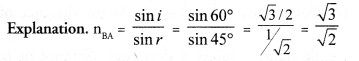Question 5.
A light ray enters from medium A to medium B as shown in figure. The refractive index of medium B relative to medium A will be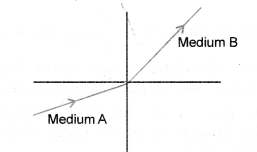(a) greater than unity
(b) less than unity
(c) equal to unity
(d) zero
(a).
Explanation :where v1 = speed of light in medium A
V2 = speed of light in medium B.
Since ray of light bends towards the normal, when it goes from medium A to rrtedium B, therefore, medium A is rarer and medium B is denser medium. Speed of light in rarer medium (v1) is greater than the speed of light in denser medium (v2). Hence, nBA > 1.

Question 6.
Beams of light are incident through the holes A and B and emerge out of a box through the holes C and D respectively as shown in figure. Which of the following could be inside the box ?(a) A rectangular glass slab
(b) A convex lens
(c) A concave lens
(d) A prism.
(a).
Explanation : Rectangular glass slab causes the lateral dis¬placement of a ray of light following on it. However, incident ray and emergent ray are paralled to each other.

Question 7.
A beam of light is incident through the holes on side A and emerges out of the holes on the other side of the box as shown in figure. Which of the following could be inside the box ?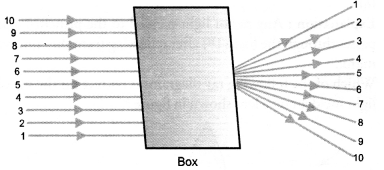(a) Concave lens
(b) Rectangular slab
(c) prism
(d) Convex lens
(d).
Explanation :Question 8.
Which of the following statements is true ?
(a) A convex lens has 4 dioptre power having focal length 0.25 m.
(b) A convex lens has – 4 dioptre power having focal length 0.25 m.
(c) A concave lens has 4 dioptre power having focal length 0.25 m.
(d) A concave lens has – 4 dioptre power having focal length 0.25 m.
(a).
Explanation : Convex lens has positive power and positive focal length.Question 9.
Magnification produced by a rear view mirror fitted in vehicles.
(a) is less than one
(b) is more than one
(c) is equal to one
(d) can be more than or less than one depending upon the position of the object in front of it.
(a).Rear view mirror is a convex mirror, which always forms an image whose size is less than the size of the object.

Question 10.
Rays from sun converge at a point 15 cm in front of a concave mirror. Where should an object be placed so that size of its image is equal to the size of the object ?
(a) 15 cm in front of the mirror
(b) 30 cm in front of the mirror
(c) between 15 cm and 30 cm in front of the mirror.
(d) more than 30 cm in front of the mirror.
(b).
Explanation : Here, Focal length of concave mirror, f = -15 cm Radius of curvature of the mirror, R = 2f = -30 cm.
In case of concave mirror, size of image is equal to the size of the object if the object is placed at the centre of curvature (see figure 20). The distance of centre of curvature from the mirror = radius of curvature of the mirror.

Question 11.
A full length image of a distance tall building can definitely be seen by using
(a) concave mirror
(b) convex mirror
(c) plane mirror
(d) both concave as well as plane mirror.
(b).
Explanation : Convex mirror forms full length of a distant tall object irrespective of the position of the object. However, plane mirror forms full size image of the object if the size of the plane mirror is half the size of the object. Concave mirror forms full size image of the object if the object is far away from it.

Question 12.
In torches, search lights and head lights of vehicles, the bulb is placed
(a) between the pole and the focus of the reflector
(b) very near to the focus of the reflector
(c) between the focus and centre of curvature of the reflector
(d) at the centre of curvature of the reflector.
(b).
Explanation :Question 13.
The laws of reflection hold good for
(a) plane mirror only
(b) concave mirror only
(c) convex mirror only
(d) all mirrors irrespective of their shape
(d).

Question 14.
The path of a ray of light coming from air passing through a rectangular glass slab traced by four students are shown as A, B, C and D in the figure. Which one of them is correct ?(B)
Explanation : Glass slab causes the lateral displacement of a ray of light falling on it. However, incident ray and emergent ray are parallel to each other.

Question 15.
You are given water, mustard oil, glycerine and kerosene. In which of these media, a ray of light incident obliquely at same angle would bend the most ?
(a) Kerosene
(b) Water
(c) Mustard Oil
(d) Glycerine
(d).
Explanation : The ray would bend the most, when it goes from rarer medium (say air) to the most denser medium. Since refrac¬tive index of glycerine is the highest among all these medium, so glycerine is the most denser medium.

Question 16.
Which of the following ray diagrams is correct for the ray of light incident on a concave mirror as shown in figure ?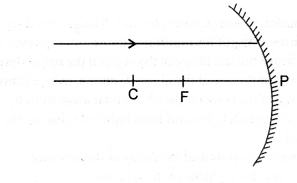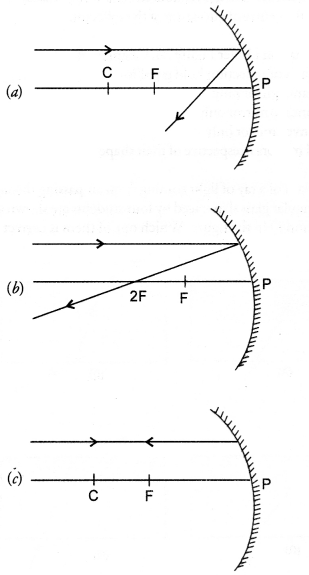(d).
Explanation : Any ray of light parallel to the principal axis passes through the focus (F) after reflecting from the concave mirror.

Question 17.
Which of the following ray diagrams is correct for the ray of light incident on a lens shown in figure ?(a).
Explanation : A ray of light passing through the focus of a lens travels parallel to the principal axis after refracting through the lens.

Question 18.
A girl is standing in front of a magic mirror. She finds the image of her head bigger, the middle portion of her body of the same size and that of the legs smaller. The order of combinations for the magic mirror from the top is :
(a) Convex, plane and concave
(b) Plane, convex and concave
(c) Concave, plane and convex
(d) Convex, concave and plane.
(c).
Explanation : Concave mirror forms a magnified (enlarged) image of the object if the object is placed close to the concave mirror (i.e. a distance less than its focal length). Plane mirror always forms the mage of the same size as that of the object.

Question 19.
Convex mirror always forms a diminished image of the object irrespective of the position of the object from the mirror. In which of the following, the image of an object placed at infinity will be highly diminished and point sized ?
(a) Concave mirror only
(b) Convex mirror only
(c) Convex lens only
(d) Concave mirror, convex mirror, concave lens and convex lens.
(d).
Explanation :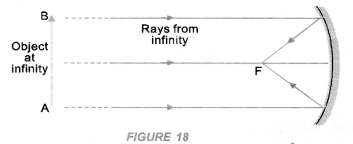Question 20.
Identify the device used as a spherical mirror or lens in the following cases, when the image formed is virtual and erect in each case.
(a) Object is placed between device and its focus, image formed is enlarged and behind it.
(b) Object is placed between the focus and device, image formed is enlarged and on the same side as that of an object.
(c) Object is placed between infinity and device, image formed is diminished and between focus and optical centre on the same side as that of the object.
(d) Obj ect is placed between infinity and device, image formed is diminished and between pole and focus, behind it.
(a) Concave mirror.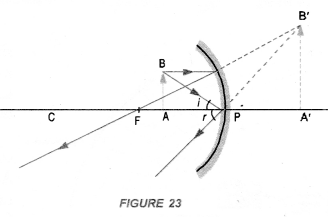(b) Convex lens.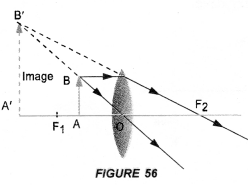(c) Concave lens.(d) Convex mirror.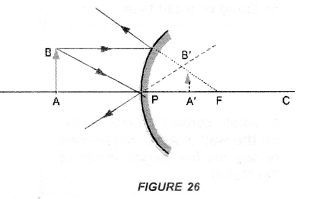Question 21.
Why does a light ray incident on a rectangular glass slab immersed in any medium emerges parallel to itself? Explain using a diagram.
Perform an experiment to demonstrate that light bends from its path, when it falls obliquely on the surface of a glass slab. Also show that angle of incidence is about equal to the emergent angle.

1. Take a glass slab and place it on a white sheet of paper fixed on a drawing board.
2. Mark the boundary ABCD of the glass slab.
3. Fix two pins P1 and P2, vertically on the drawing board such that line joining the pins is inclined to the edge AB of the glass slab (Figure 37).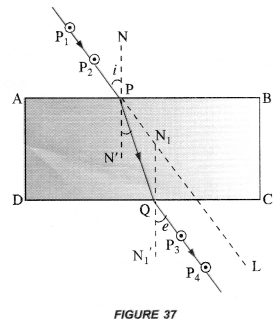4. Now, look through the glass slab from opposite side so that the images of pins P1 and P2 are seen exactly in line. Fix two pins P3 and P4, vertically on the drawing board such that pins P3, P4 and image of P1 and P2 are exactly in one line.
5. Remove the glass slab and all pins. Join the points P1 and P2 with a line and extend this line to touch the edge AB at P. Similarly join the points P3 and P4 with a line and extend the line to touch the edge DC at Q.
6. Join points P and Q with a straight line.
7. Draw normal NN’ at P on the edge AB and a normal N1N1‘ at Q on the edge DC.
8. Measure ∠P2 PN and ∠P3 QN1‘ using a protractor or dee.

This experiment shows that when light falls obliquely on a glass slab, it bends along PQ from its original path along PL.
Also ∠P2PN ≈ ∠P3QN1‘. That is, angle of incidence is about equal to the emergent angle.
Note : Distance between pins P1 and P2, and between P3 and P4 must be large.

Question 22.
A pencil when dipped in water in a glass tumbler appears to be bent at the interface of air and water. Will the pencil appear to be bent to the same extent, if instead of water, we use liquids like, kerosene or turpentine. Support your answer with reason.
A pencil dipped in water appears to be bent at the interface of air and water due to the refraction of light. The refraction of light occurs because the speed of light changes when light travels from one medium to another. The pencil will not appear to be bent to the same extent, when it is dipped in kerosene or turpentine. This is because refractive index of kerosene or turpentine is greater than the index of water and hence speed of light (v = c/n) is less in kerosene or turpentine as compared to in water.

Question 23.
How is the refractive index of a medium related to the speed of light ?
Obtain an expression for refractive index of a medium with respect to another in terms of speed of light in these two media.
Refractive index of a medium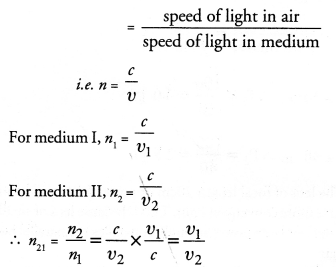Question 24.
Refractive index of diamond with respect to glass is 1.6 and absolute refractive index of glass is 1.5.
Find out the absolute refractive index of diamond.Question 25.
A convex lens of focal length 20 cm can produce a magnified virtual as well as real image.
Is this a correct statement ? If yes, where shall the object be placed in each case for obtaining these images ?
The statement is correct.
A convex lens of focal length 20 cm will produce a magnified Virtual image if object is placed at a distance less than 20 cm from the lens.A convex lens of focal length 20 cm will produce a magnified real image if object is placed at a distance greater than 20 cm and less than 40 cm from the lens.Sudha finds out that the sharp image of the window pane of her science laboratory is formed at a distance of 15 cm from the lens. She now tries to focus the building visible to her outside the window instead of the window pane without disturbing the lens.

Question 26.
In which direction will she move the screen to obtain a sharp image of the building ? What is the approximate focal length of this lens ?
A real image can be obtained on the screen. Therefore, the lens used is convex lens as it forms real as well as virtual image. The distance of the real image formed by a convex lens from the lens decreases as the object distance from the lens increases. Hence, the screen has to be moved towards the lens to obtain the sharp image of the building.
Approximate focal length of the lens = 15 cm. The rays of light from the window pane are considered to come from infinity. These rays of light are focused by the convex lens at its focus (i.e. on the screen).

Question 27.
How are power and focal length of a lens related ? You are provided with two lenses of focal length 20 cm and 40 cm respectively. Which lens will you use to obtain more convergent light ? (CBSE 2012)The lens of focal length 20 cm or power 5.0 D will be used to have more convergent light. This is because lens of small focal length or large power strongly converges the parallel beam of light.

Question 28.
Under what condition in an arrangement of two plane mirrors, incident ray and reflected ray will always be parallel to each other, whatever may be the angle of incidence. Show the same with the help of diagram.
Incident ray and reflected ray will always be parallel to each other if two plane mirrors are placed perpendicular to each other as shown in figure, i = r.Question 29.
Draw a ray diagram showing the path of rays of light when it enters with oblique incidence

1. from air into water,
2. from water into air.